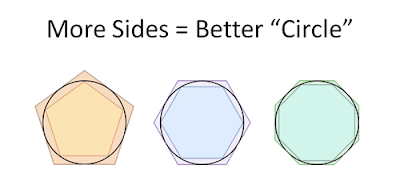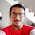# The Wonderful π

We are surrounded by atoms, molecules, clocks, balls, planets, the Sun, and the list goes on. All the above objects or matter are considered circular in 2-dimensions. It is easier to measure the length of a line using a ruler. The circumference of a wall clock can be measured by tracing a string around the clock and measuring the distance between endpoints using a ruler.

Back in the stone age, the wheelbarrow was used to transport goods to different locations. The wheel attached to it was not circular enough, which made a troublesome experience in transportation. Measurement came into the mind of people that a perfectly circular wheel must have a diameter of 7 units and a circumference of 22 units.

# What is Pi?

Pi is the 16th alphabet of Greek literature same as P, the 16th alphabet of English literature. Mathematicians have found out when the ratio of circumference to the diameter of a circle is taken, irrespective of the size of the circle, the answer is always Pi.Source: Nasa

The value of Pi is estimated to be about 3.141592653589793238 up to 18 decimal digits or simply 22/7 in-fraction. If we cut several pieces of string equal in length to the diameter, we will need a little more than three of them to cover the circumference of the circle.Source: Amazing Archimedes

In the ancient world, Babylonians, Egyptians, Greeks, Chinese and Indian mathematicians had estimated the value of Pi using different methods. The first calculation of π was done by Archimedes of Syracuse (287–212 BC). He draws the polygon inscribed within the circle and the polygon within which the circle was circumscribed.

He could only find the approximate value of Pi within close limits. With a similar approach Zu Chongzhi (429–501 BC), a Chinese mathematician and astronomer came with the ratio of circumference to the diameter of a circle as 355/113.

To date, the exact value of Pi is still a mystery, cannot be placed on a number line. The value of Pi goes on...... to make it an irrational number. The fraction 22/7 = 3.142857 is accurate up to 2 decimals only and 355/113 = 3.14159292035 is only accurate up to 8 decimals. Although  3.14   is sufficient enough for simple calculations.

The most accurate value of Pi up to 50,000,000,000,000 digits (50 trillion) was found out by Timothy Mullican in Alabama, United States on 29th January 2020 and recorded himself in the Guinness Book of World Records. He ran Chudnovsky's algorithm on the supercomputer and took 8 months in total.

# Uses of Pi

In basic mathematics, Pi is used to find the area and circumference of a circle. For building and construction, quantum physics, communications, medical procedures, air travel, etc., every estimation is incomplete without Pi. NASA regularly uses it in calculating trajectories of spacecraft, the size of craters, and dimensions of extrasolar planets. The size of an atom, periodic and oscillating systems like pendulums, electromagnetic waves, and even music theory are explained using Pi. In general, the application of Pi is limitless.

# Fun facts

International Pi day is celebrated on the 14th of March every year. Why this day? Considering Pi = 3.14  , the 14th day of the 3rd month of the year, i.e. 14th March is taken.

Famous German Physicist Albert Einstein was born on 14th March 1979 and well-known English theoretical Physicist Stephen Hawking (remembered for his book A Brief History of time) passed away on 14th March 2018 at the age of 76, which is a strange coincidence.

Where there is Mathematics, there will be a Pi.

1.1.2.1.3.1.